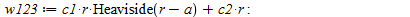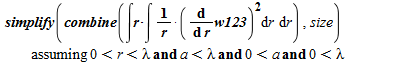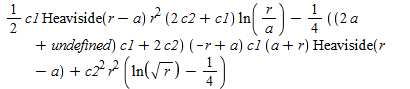## How to create all possible lists containing m elem...

Hey everyone,

New here but I'm a bit stuck. Ive looked around in list tools as well as tried but I havent had any luck.

Suppose I have a list defined as FirstList:=[A,B,C,D,E,F]. Now I want to create (and label) all possible
lists from FirstList which contain 4 elements which dont have an element repeated within the same list
In this example, there are 15 lists in total i.e.

Sublist:=[A,B,C,D]:
Sublist:=[A,B,C,E]:
Sublist:=[A,B,C,F]:
Sublist:=[A,C,D,E]:

..etc

Is there a simple way to do this???

Any help is appreciated!

## Executing Sections in Maple...

Is there away to quickly execute a specific section within Maple? I know that I can execute a selection, but that sometimes requires me to select several lines of Maple code and can be tedious and tiresome.

## Build array of coordinates from implicit equation....

Hallo,

Is there a fairly straightforward method for obtaining an array of coordinates from an implicit equation? I have an ellipse defined implicitly (by a horrendously involved expression) and can't figure out how to extract a set of coordinates from an implicitplot. I'm reluctant to use seq and fsolve with a fixed stepsize.

Would be grateful for some insight!

Thanks.

Weirp

## How do I plot 2D animate in Maple?...

I wish to  plot 2D animate for the soltion of this equation here is the code

restart;
with(PDEtools):
with(ArrayTools):
with(plots):

f:=u->sech(u):
g:=v->sech(v):
h:=1/10:
N:=20:
M:=20:
V:=x->x^2:
psi:=Array(0..N/h+1,0..M/h+1):
for i from 0 to N/h do
psi[i,0]:=evalf(f(i*h)):
od:
for j from 0 to M/h do
psi[0,j]:=evalf(g(j*h)):
od:
for i from 1 to N/h do
for j from 1 to M/h do
psi[i,j]:=-psi[i-1, j-1]+(1-(1/8)*h^2*V((1/2)*h*(j-i-1)))*psi[i, j-1]+(1-(1/8)*h^2*V((1/2)*h*(j- i+1)))*psi[i-1,j]:
od:
od:
ls:=[seq([seq([i*h,j*h,psi[i,j]],i=0..N/h)],j=0..M/h)]:## OpenMaple Problem...

Hello,

I am writing a program in C that uses the open maple library. It is not the first time that I use it but now I am facing a strange problem that involves the simplify command: suppose a,x,y are symbols that are not previously used in maple, the following lines

1)  EvalMapleStatement(kv, "simplify((a*x^2-y^2)/(x^2*y^2-1));");

2)  EvalMapleStatement(kv, "simplify((2*x^2-y^2)/(x^2*y^2-1));");

only differ by the fact that the parameter a is replaced by 2 in the second line. But they return the following output:

1) (a*x^2-y^2)/(x^2*y^2-1)

that is correct, nothing to simplify..

2) Error, (in gcd/LinZip) input must be polynomials over the integers

I must be doing something wrong but I am getting nowhere...

Thanks...

P.S. This is the complete listing

#include <stdio.h>

#include <stdlib.h>

#include "maplec.h"

static void M_DECL textCallBack( void *data, int tag, char *output )

{

printf("%s\n",output);

}

int main( int argc, char *argv[] )

{

char err;  /* command input and error string buffers */

MKernelVector kv;  /* Maple kernel handle */

MCallBackVectorDesc cb = {  textCallBack,

0,   /* errorCallBack not used */

0,   /* statusCallBack not used */

0,   /* readLineCallBack not used */

0,   /* redirectCallBack not used */

0,   /* streamCallBack not used */

0,   /* queryInterrupt not used */

0    /* callBackCallBack not used */

};

ALGEB r, l;  /* Maple data-structures */

char *myargv[]={"maple"};

int myargc=1;

if( (kv=StartMaple(myargc,myargv,&cb,NULL,NULL,err)) == NULL ) {

printf("Fatal error, %s\n",err);

return( 1 );

}

EvalMapleStatement(kv, "simplify((a*x^2-y^2)/(x^2*y^2-1));");

EvalMapleStatement(kv, "simplify((2*x^2-y^2)/(x^2*y^2-1));");

StopMaple(kv);

return( 0 );

}

compiled with

gcc prova.c -I /Library/Frameworks/Maple.framework/Versions/Current/extern/include/ -L /Library/Frameworks/Maple.framework/Versions/Current/bin.APPLE_UNIVERSAL_OSX/ -l maplec

## Saving the solution from fsolve...

Hello,

I have a question. Consider

fsolve(x^2+3*x+1=3, x);

I want to save the two roots into two variables. What kind of commend shall I use?

P.S. My further aim comes from solving an equation without analytical solution. Therefore I cannot plug in the solution formula.

## matrix multiplication element by element...

Who knows: is there a maple command for two matrix multiplication element by element without summing?

## Error in 2nd Order Coupled Nonlinear ODE System...

I'm calculating the geodesics to a parametrized system in R3. When trying to solve the geodesic equations for a surface of revolution, I'm getting a strange error. The goal is to write the code for any parametrized surface, not just revolution (hence I didn't use the short cut for surfaces of revolution).

For the code, I find the first fundamental for, then the geodesics, then turn the christoffel symbols into a time-variant array. (Gamma for the position of (u,v) in the uv-plane, and C as the array so I can take derivatives with respect to time.)

The code for creating the Christoffel Symbols and the parametrization of the paraboloid:

restart; with(LinearAlgebra):
V := (u, v) -> <v*cos(u), v*sin(u), v^2+1>;

Christoff := proc (X)
local x1, x2, M, N, i, j, k, s, E, F, G, g, Q, Delta, Prelim, cyclicPrelim;
global Gamma, C; #GAMMA
x1 := (u, v) -> <diff(X, u), diff(X, u), diff(X, u)>;
x2 := (u, v)-> <(diff(X, v), diff(X, v), diff(X, v))>;
E :=  (u, v) -> DotProduct(x1(u, v), x1(u, v), conjugate = false);
F := (u, v) -> DotProduct(x1(u, v), x2(u, v), conjugate = false);
G := (u, v) -> DotProduct(x2(u, v), x2(u, v), conjugate = false);
simplify([E(u, v), F(u, v), G(u, v)]);
M := (u, v) -> <E(u, v), F(u, v); F(u, v), G(u, v)>;
simplify(M(u,v));
printlevel := 3;
Delta := simplify(Determinant(M(u, v)));
N := (1/Delta)*<G(u, v), -F(u, v); -F(u, v), E(u, v)>;
Q := simplify(map(diff, M(u, v), u));
Q := simplify(map(diff, M(u, v), v));
for i to 2 do for j to 2 do for k to 2 do
Prelim[i, j, k] := Q[k][i, j];
simplify(Prelim[i, j, k] ); end do end do end do;
#(OPTIONAL PRINTOUT) print(Prelim);
for i to 2 do for j to 2 do for k to 2 do
cyclicPrelim[i, j, k] := Prelim[i, j, k]+Prelim[j, k, i]-Prelim[k, i, j] ;
end do end do end do;
#(OPTIONAL PRINTOUT)  print(cyclicPrelim);
for i to 2 do for j to 2 do for k to 2 do
Gamma[i, j, k] := simplify((1/2)*add(N[i, s]*cyclicPrelim[j, s, k], s = 1 .. 2));
end do end do end do;
# for k from 1 to 2 do
#print(`GAMMA[i,j,k] =` Matrix([[ `%a` , `%a` ],[ `%a` , `%a` ]])` \n`, Gamma[1,1,k], [Gamma[1,2,k], Gamma[2,1,k], Gamma[2,2,k]);
# end do;
#printf('GAMMA[%a,%a,%a] = %a \n', i,j,k, Gamma[i,j,k]);

print([Gamma[1,1,1], Gamma[1,2,1], Gamma[2,1,1], Gamma[2,2,1], Gamma[1,1,2], Gamma[1,2,2], Gamma[2,1,2], Gamma[2,2,2]]);

for i from 1 to 2 do
for j from 1 to 2 do
for k from 1 to 2 do
C[i,j,k]:= apply(Gamma[i,j,k],t);
end do end do end do;
C:=Array(1..2,1..2,1..2,[ [ [ apply(Gamma[1,1,1],t), apply(Gamma[1,2,1],t) ], [ apply(Gamma[1,1,2],t), apply(Gamma[1,2,2],t) ] ], [ [ apply(Gamma[2,1,1],t), apply(Gamma[2,2,1],t) ],  [ apply(Gamma[2,1,2],t), apply(Gamma[2,2,2],t) ] ] ]);
print(C);

end proc;

The differential equation solver:

inits:=[u(0)=1, D(u(t))(0)=1,v(0) = 1, D(v(t))(0)=1];

sys1:= [D[1\$2](u(t))+C[1,1,1]*(D(u(t)))^(2)+2*C[1,1,2]*(D(u(t)))*(D(v(t)))+C[1,2,2]*(D(v(t)))^2=0, D[1\$2](v(t))+C[2,1,1]*(D(u(t)))^(2)+2*C[2,1,2]*(D(u(t))*D(v(t)))^(2)+C[2,2,2]*(D(v(t)))^2=0];

L:=dsolve({sys1} union {inits});

The error message that comes up is:

"Error, (in unknown) invalid input: op expects 1 or 2 arguments, but received 0"

Any help would be greatly appreciated.

## How to get a printable Maple 16 Quick Reference Ca...

I have Maple 16 for Windows 64-bit. How to get a printable Maple 16 Quick Reference Card? Also, how to save the Maple 16 Quick Reference Card as a separate file? (When I try to copy the Card I get en error message "selection too large").

## Problems with limit cycles and maple 16 ...

I am having trouble printing out a limit cylce on maple 16.  I have the attached file and if anybody could look at it and perhaps help me out it would be greatly appreciated.  The first limit cycle is supposed to look somewhat like the second one.  I'v tried many different things but nothing seems to be working.  an explenation would also be nice too.  if the file does not open correctly also let me know. thank you very much.

## How can I do an animation?...

Hi,

I want to animate this code

plots[odeplot](Trajectoire, [[x1(t), y1(t), t], [x2(t), y2(t), t], [x3(t), y3(t), t], [x4(t), y4(t), t], [x5(t), y5(t), t], [x6(t), y6(t), t], [x7(t), y7(t),t], [x8(t), y8(t), t], [x9(t), y9(t), t], [xA(t), yA(t), t]], TempsInitial..TempsFinal, numpoints = 10000,axes=boxed, scaling = constrained);

However, Maple 16 is giving me an error message :

Error, (in plots/animate) the first argument must be a procedure

I don't know how I can do to animate this code.

Thanks!

Hello,

sometimes I observe extreme delays when simply entering a variable name like fooBar; right after starting a Maple session. This happens when the current directory contains a file with that name, because Maple automatically loads this file.

For example, if I create an empty file fooBar.m and start a Maple session,

`> fooBar;Error, `./fooBar.m` is an incorrect or outdated .m file`

raises an error. More often however, I have some huge Maple files in the working directory and whenever I use (by chance) a variable name in the Maple session that matches a filename, Maple tries to load the corresponding file.

Is there a documentation of this behaviour and a possibility to stop this automatic loading of files?

Thanks,

Erik

## Heaviside successive integration with undefined in...

Dear All,

I would like perform a symbolic integration to the following integrand with Heaviside function:The integral I defined is as follows:The output I got isCould any one explain me how to avoid the undefined in the output. I tried to define the assumtions to constrain the solution. However, I failed to supress the undefined.

I expect a result of the integration should also be piecewise function.

Appreciate you constructive inputs.

Thanks!

## Why are polynomials including constants not factor...

Hello,

I have trouble in using the function factors. For example, I expect

`factor(Pi*(t^2+1), {I});`

to output

`-Pi*(-t+I)*(t+I)`

`Pi*(t^2+1)`

This problem does not appear if Pi gets replaced by a general symbol:

`factor(pi*(t^2+1), {I});`

produces (as I expect it should)

`-pi*(-t+I)*(t+I)`

The problem seems to be tied to symbols representing constants, as for example replacing Pi by Catalan also results in no factorization being performed. It further seems to be tied to specifying a splitting field, because

`factor(Pi*(t^2-1));`

results in

`Pi*(t-1)*(t+1)`

Is this behaviour intended? Probably the reason is that the polynomial does not have algebraic coefficients (as it includes Pi). Indeed,

`factor(Pi*(t^2-1),{});`

produces the error message

`Error, (in factor) expecting a polynomial over an algebraic number field`

But why does this error then not appear for the call factor(Pi*(t^2-1))? If this would assume complex coefficients, it should factor using I. Considering coefficients in an algebraic number field, also the original call factor(Pi*(t^2+1), {I}); should raise an error!?

Thanks,

Erik

## how to fix {Error, (in DEtools/DEplot/direction) d...

I have tried to use Maple to solve ordinary differential equations but i have this error. Could you please help me to fix this problem

> eq1 := diff(v1(t), t) = v2(t);
> eq2 := diff(v2(t), t) = -v1(t)+(3*(v1(t)^2-1))*v2(t);
> init1 := v1(0) = 2;
> init2 := v2(0) = 0;
> with(DEtools);
> DEplot({eq1, eq2}, [v1(t), v2(t)], 0 .. 3*Pi, {[0, 0, 0]}, scene = [v1, v2], stepsize = .1);

Error, (in DEtools/DEplot/direction) division by zero

thank you

﻿